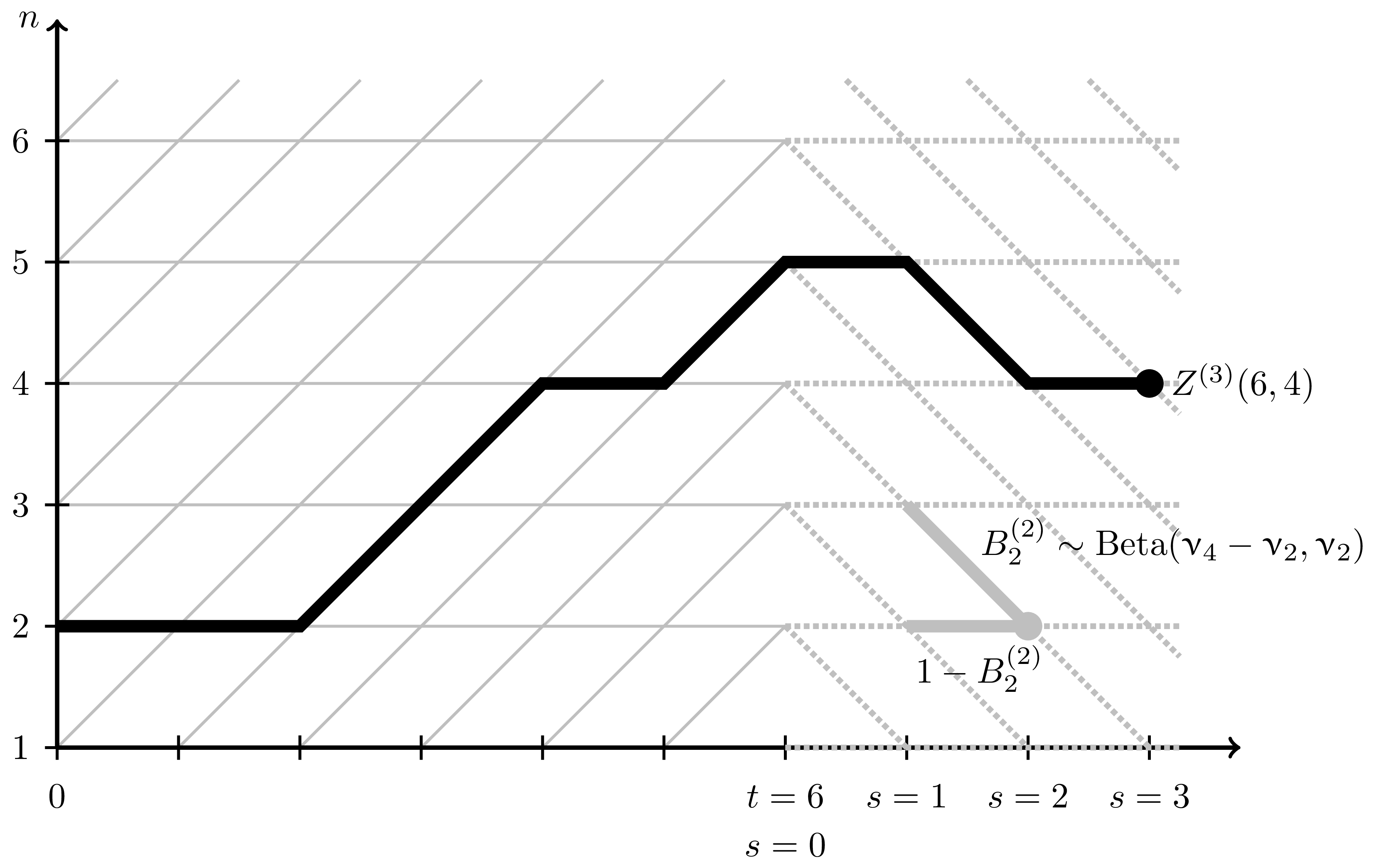# Parameter permutation symmetry in particle systems and random polymers

### 2019/12/12

arXiv:1912.06067 [math.PR]

PDF

Many integrable stochastic particle systems in one space dimension (such as TASEP - Totally Asymmetric Simple Exclusion Process - and its various deformations, with a notable exception of ASEP) remain integrable when we equip each particle $x_i$ with its own jump rate parameter $\nu_i$. It is a consequence of integrability that the distribution of each particle $x_n(t)$ in a system started from the step initial configuration depends on the parameters $\nu_j$, $j\le n$, in a symmetric way. A transposition $\nu_n \leftrightarrow \nu_{n+1}$ of the parameters thus affects only the distribution of $x_n(t)$. For $q$-Hahn TASEP and its degenerations (namely, $q$-TASEP and beta polymer) we realize the transposition $\nu_n \leftrightarrow \nu_{n+1}$ as an explicit Markov swap operator acting on the single particle $x_n(t)$. For beta polymer, the swap operator can be interpreted as a simple modification of the lattice on which the polymer is considered. Our main tools are Markov duality and contour integral formulas for joint moments.

In particular, our constructions lead to a continuous time Markov process $\mathsf{Q}^{(\mathsf{t})}$ preserving the time $\mathsf{t}$ distribution of the $q$-TASEP (with step initial configuration, where $\mathsf{t}\in \mathbb{R}_{>0}$ is fixed). The dual system is a certain transient modification of the stochastic $q$-Boson system. We identify asymptotic survival probabilities of this transient process with $q$-moments of the $q$-TASEP, and use this to show convergence of the process $\mathsf{Q}^{(\mathsf{t})}$ with arbitrary initial data to its stationary distribution.

Setting $q=0$, we recover the results about the usual TASEP established recently in this paper by a different approach based on Gibbs ensembles of interlacing particles in two dimensions.Modification of the lattice leading to the elimination of parameters of the beta polymer